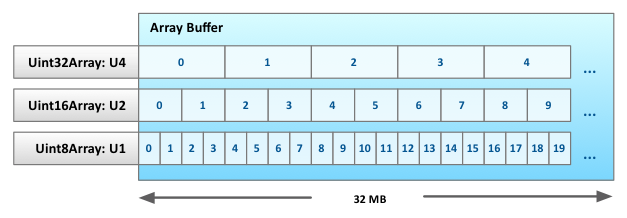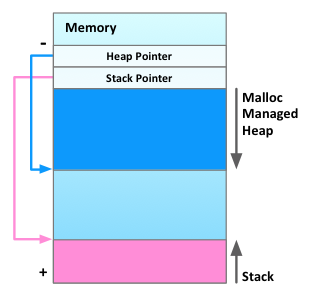# LLJS : Low-Level JavaScript

LLJS is a typed dialect of JavaScript that offers a C-like type system with manual memory management. It compiles to JavaScript and lets you write memory-efficient and GC pause-free code less painfully, in short, LLJS is the bastard child of JavaScript and C. LLJS is early research prototype work, so don't expect anything rock solid just yet. The research goal here is to explore low-level statically typed features in a high-level dynamically typed language. Think of it as inline assembly in C, or the unsafe keyword in C#. It's not pretty, but it gets the job done.

This is an interactive tutorial, code is compiled as you type. To execute a piece of code press Ctrl-Enter or Cmd-Enter.

## Variables

Unlike JavaScript, LLJS variable declarations are block scoped (only the let keyword is allowed) and can be annotated with type information. Untyped variable declarations are defaulted to the dyn type.

```extern timer;

let x;               // Declare 'x' as dyn.
let int y;           // Declare 'y' as int.

y = (int)(x);        /* Assignment of 'x' to 'y' requires an explicit
cast. */

let int z = y + 1;   /* Although 'y' is of type int, the binary
expression y + 1 is of type num and
requires an implicit cast. */

let uint w = z;      /* Unsigned integer types are supported but
are discouraged because JavaScript engines
usually store numbers that are larger than
max signed int in doubles rather than 32-bit
ints. */

timer.begin("Empty For loop with signed integers.");
for (let int i = 0; i < 50000000; ++i) { }

timer.begin("Empty For loop with unsigned integers.");
for (let uint i = 0; i < 50000000; ++i) { }

timer.begin("Empty For loop with untyped integers.");
for (let i = 0; i < 50000000; ++i) { }```

## Data Types and Arithmetic

LLJS has 8 numeric types: i32 (int), u32 (uint), i16, u16, i8, u8, float and double which behave as they do in C, and two additional types: num (the JavaScript number type) and dyn (any type) which are used to interoperate with the JavaScript type system.

```trace("    u8: " + sizeof (u8));
trace("    i8: " + sizeof (i8));
trace("   u16: " + sizeof (u16));
trace("   i16: " + sizeof (i16));
trace("   u32: " + sizeof (u32));
trace("   i32: " + sizeof (i32));
trace(" float: " + sizeof (float));
trace("double: " + sizeof (double));

trace(" u8: " + (u8)(-1));
trace(" i8: " + (i8)(-1));
trace("u16: " + (u16)(-1));
trace("i16: " + (i16)(-1));
trace("u32: " + (u32)(-1));
trace("i32: " + (i32)(-1));

let int x = 3;
let int y = 2;

/* Arithmetic follows C semantics. Arithmetic on integers begets
integers, truncated with | 0. */
trace("Result is an integer: " + x / y);
trace("Integral literals are typed as integers: " + 1 / 2);
trace("Floating point literals are typed as double: " + 1.1 / 2);
```

Moreover, LLJS lets you define your own struct, union and pointer types.

```let int x = 42;      // Declare 'x' as int and assign 42 to it.
let int *y = &x;     /* Declare 'y' as a pointer to int and assign it
the address of x. Since JavaScript doesn't
allow taking references to variables, we
allocate 'x' on an emulated stack. */
let int **z = &y;    // Declare 'z' as a pointer to a pointer to int.
trace(x);
*y = 1;              // Assign to the variable pointed to by 'y'.
trace(x);
**z = 12;
trace(x);
**z = ***(&z);       // You can get as fancy as you want.
```

Structs are defined using the struct keyword.

```struct Point {
int x, y, z;
};

struct Line {
Point start, end;
};

struct Box {
Line left, top, right, bottom;
};

let Box b;
trace(b.top.start.x);

let Line *p = &b.top;
p->start.x = 42;
trace(b.top.start.x);```

Similarly, unions are defined using the union keyword.

```union Box {
int i;
float f;
};

let Box box;
box.f = 123.456;
trace(box.i);        // Read back box.f as an integer.
```

#### Functions

Function declarations can be typed, a typed function is any function that has its return value or at least one of its arguments typed.

```
// Declare 'foo' as dyn, normal JavaScript function.
function foo() { }

// Declare 'bar' as () -> void, typed LLJS function.
function void bar() { }

// Declare 'baz' as (int, int*) -> void, typed LLJS function with typed
// arguments.
function void baz(int x, int *y) { }

// Declare 'quux' as (int, dyn, int x) -> dyn, typed LLJS function with
// mixed typed and untyped arguments.
function quux(int x, y, int z) { }

// Call 'quux'. Argument passing follows the same typechecking rules as
// assignment.
quux(-123, 2, 123.456);

// The type system is not smart enough to track types that leak into
// the dynamic type system. Safety is your responsibility.
let unsafeQuux = quux;

// Calling 'unsafeQuux' is not type-safe.
unsafeQuux("123", 2, 123.456);```

Example: you can implement a swap function in LLJS as follows:

```function void swap(int *a, int *b) {
let int t = *a;
*a = *b;
*b = t;
}

let int x = 1, y = 2;

swap(&x, &y);

trace("x = " + x + ", y = " + y);```

#### Arrays

Typed arrays can be allocated on both the heap and the stack. Stack allocated arrays must, as in C, be fixed length. Heap allocated arrays use a similar syntax to C++ dynamic arrays and their size may be computed at runtime.

```
// Stack allocated, fixed size
let int arr;
arr = 42;
trace(arr);

// Heap allocated, dynamic size
for (let int i=1; i <= 10; i++) {
let int *heapArr = new int[i];
arr[i-1] = i;
trace(i + ": " + arr[i-1]);
}

// Stack allocated array inside a struct
struct ArrayStruct {
int arr;
};

let ArrayStruct s;
s.arr = 42;
trace(s.arr);
```

## Objects and Memory

LLJS has two object models: C style malloc and free, and the JavaScript object model. Why would you ever want to manage your memory explicitly when the JavaScript garbage collector already does the work for you. Well imagine you wanted to write a linked list in JavaScript. You would probably chain a sequence of objects together, like so:

``` var head = {value: 0, next: null}, tail = head;

var next = {value: value, next: null};
tail.next = next;
tail = next;
}
```

This is inefficient for several reasons. Objects in JavaScript are not cheap, they need to carry around lots of extra information and can be many times larger than their C style counterparts, moreover property access can be slow. Chaining several property accesses together x.y.z.w usually results in several memory indirections, in LLJS this compiles into a single memory access with a computed offset.
Usually, when you use a garbage collector you can design a very fast bump allocator which is probably more efficient than LLJS's malloc, but nothing is free, you pay for it later during garbage collection. If you have explicit control over memory, you can always do better (if you put in the effort). In LLJS you can write a much more space efficient linked list using pointers and structs.

```struct Node {
Node *next;
int value;
};

let Node *next = new Node;
next->value = value;
tail->next = next;
tail = next;
return next;
}

function void traceList(Node *p) {
while (p) {
trace("Node at address: " + p + ", has value: " + p->value);
p = p->next;
}
}```

You may notice that the new operator behaves differently whenever it's applied to a type name. It computes the size of the data item in bytes, and calls malloc.

```// Import malloc. The casting is annoying -- we don't have a good
// story for typed imports yet.
typedef byte *malloc_ty(uint);
let malloc_ty malloc = (malloc_ty)(require('memory').malloc);

// Allocate an int on the heap using new.
let int *y = malloc(sizeof(int));
trace(y);

// Alternate, more convenient syntax.
let int *x = new int;
trace(x);```

### Memory

LLJS manages memory using Typed Arrays. It allocates one large ArrayBuffer ahead of time, and creates several typed array views on this buffer, one for each of the primitive data types. For instance: U4, U2 and U1 for u32, u16 and u8 respectively (see figure below). (For a more in-depth discussion, see how Emscripten does it.) This really is the same thing as the scaled/indexed addressing mode in x86. The only drawback is that you can't read unaligned data, but this is actually a good thing, since unaligned access is usually slower, or not even possible on some platforms.### Pointers

Since our managed memory is actually addressable at byte and integer levels, there is no reason to store pointers as byte addresses. Instead we store them as indices, implicitly scaled by their own base type. For example, the pointer dereference *p, where p is of type int*, gets compiled into U4[p]. Had we stored pointers as byte addresses, we would have compiled it to U32[p >> 2]. This eliminates address computations at each memory access, but requires that we perform pointer conversions whenever we cast from one pointer type to another, see below:

```let int *x = (byte *)(8);
let u16 *y = x;
let int z = *x + *y;```

### Malloc & Free

The LLJS memory allocator, transcribed from the K&R C implementation, is itself implemented in LLJS. For now, we allocate one large chunk of memory 32 MB and let malloc and free manage it. We reserve the first two words in memory for the Heap Pointer HP that points to the latest allocated page by sbrk and the Stack Pointer SP which always points to the top of the stack. The heap grows downward (towards higher addresses) and the stack grows upward. For now, when the two meet we run out of memory, but we could resize the array buffer copy the heap over and resume.### Contrived Allocation Benchmark

Here is a contrived allocation benchmark that compares allocating linked list nodes with the slow K&R malloc versus using native JavaScript objects. We allocate and free 250,000 structs of 40 bytes each and compare it against allocating 250,000 objects with 4 extra properties. The struct version is much more space efficient, and as we will see, is at least as good performing, if not better, than allocating objects.

At the time of this writing, allocating structs is about as fast as allocating objects on v8, but noticeably faster on SpiderMonkey.

```struct Node {
Node *next;
int val;
};

function ObjNode() {
this.next = null;
this.val = 0;
}

extern Date;

const int n = 250000;

function benchStruct() {
let start = new Date();
for (let int i = 0; i < n; ++i) {
}

let double sum = 0;
for (let int i = 0; i < n; ++i) {
delete prev;
}

return new Date() - start;
}

function benchObject() {
let start = new Date();
for (let i = 0; i < n; ++i) {
}

let sum = 0;
for (let i = 0; i < n; ++i) {
}

return new Date() - start;
}

// Warm up the JIT.
benchStruct(); benchStruct();
benchObject(); benchObject();

let dt;

dt = 0;
for (let i = 0; i < 10; ++i) {
dt += benchStruct();
}
trace("Structs: " + dt / 10 + " ms");

dt = 0;
for (let i = 0; i < 10; ++i) {
dt += benchObject();
}
trace("Objects: " + dt / 10 + " ms");
```

#### Acknowledgements

Emscripten for the inspiration, Esprima and Escodegen for parsing and code generation, and CodeMirror for the code editor.